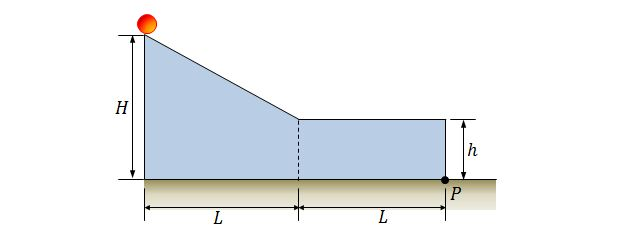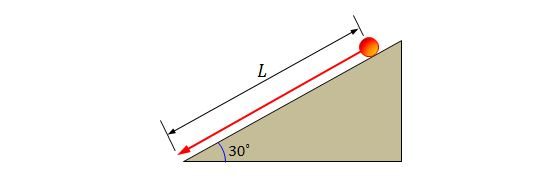Classical Mechanics

# Conservation of rotational and translational energyA solid ball rolls on a slope from rest starting from a height of $H=7.0\text{ m}$ and then rolls on a horizontal region, as shown in the above figure. The horizontal distance of the slope and the distance of the horizontal region are both equal to $L=6 \text{ m},$ and the height of the horizontal region is $h=3.0\text{ m}.$ Approximately how far horizontally from point $P$ does the ball hit the floor?

The rotational inertia of a solid sphere about any diameter is $I=\frac{2}{5}MR^2,$ where $M$ and $R$ are the mass and the radius of the solid sphere, respectively, and the gravitational acceleration is $g=9.8\text{ m/s}^2.$

A brand new off-road $6 \times 6$ pickup truck has six wheels. If the total mass of the pickup truck is $1200\text{ kg}$ and the mass of each of the six wheels is $19\text{ kg},$ what fraction of its total kinetic energy is due to the rotation of the wheels about their center axles?

Assume that each of the six wheels is a uniform disk.

A very thin hoop with a mass of $180\text{ kg}$ is rolling along a horizontal floor. If the speed of the hoop's center of mass is $0.180\text{ m/s},$ how much work must be done on the hoop to stop it?A solid cylinder with radius $40\text{ cm}$ and mass $12\text{ kg}$ is rolling down from rest without slipping for a distance of $L=10.0\text{ m},$ as shown in the above figure. The angle of the slope is $\theta=30^\circ.$ What is the approximate angular speed of the cylinder about its center when it just meets the bottom of the slope, assuming that the gravitational acceleration is $g=9.8\text{ m/s}^2?$

Consider a situation where a uniform solid sphere, which was rolling smoothly along a horizontal floor, rises up along a $30.0^\circ$ slope. If it stops after it has rolled $9.00\text{ m}$ along the slope and then begins to roll backward, what was its initial speed?

The rotational inertia of a solid sphere about any diameter is $I=\frac{2}{5}MR^2,$ where $M$ and $R$ are the mass and the radius of the solid sphere, respectively, and the gravitational acceleration is $g=9.8\text{ m/s}^2.$

×